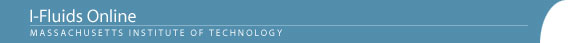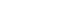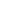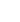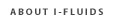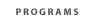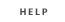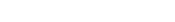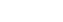2.25: Advanced Fluid Dynamics Section 1: Continuum viewpoint and the equation of motion Section 2: Static Fluids Section 3: Mass Conservation Section 4: Inviscid flow - differential approach Section 5: Control Volume Theorums Section 6: Navier-Stokes equation and viscous flow Section 7: Similarity and dimensional analysis Section 8: Boundary layers, separation and effect on drag/lift Section 9: Vorticity and circulation Section 10: Potential flows; lift, drag and thrust production Section 11: Surface tension and its effect on flows Section 12: Introduction to turbulence Back to 2.25 HomeSection 3: Mass Conservation of Flowing Media3.1 Law of mass conservation for a continuum, expressed in control volume form. Examples.
3.2 Mass conservation law in differential form. The physical significance of the divergence of the velocity: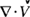= the rate of increase of the material’s volume, per unit volume.
3.3 Some special forms of the mass conservation equation for quasi-one-dimensional flow, accounting for the effects of unsteadiness, compressibility, and cross-sectional area variations. Examples.

Reading

Fay, Chapter 3

Problem Set Section 3
Problem 3.3
Problem 3.5
Problem 3.7
Problem 3.8